## Diffusion (ImageXd)

Diffusion allows the mapping of channels or structures (here biological tissues). It creates a vector field based on all voxels and its surroundings.

First used in Image3d for:
Kupczik K, Stark H, Mundry R, Neininger F, Heidlauf T, Röhrle O (2015). Reconstruction of muscle fascicle architecture from iodine-enhanced microCT images: a combined texture mapping and streamline approach. Journal of Theoretical Biology 382 (7): 34–43. DOI:10.1016/j.jtbi.2015.06.034 / ISSN:0022-5193

In this example, we use a part of an image stack from Julian Sartori (Thanks for the support!), which shows the achilles tendon of a rat.

Download: Image stack

To convert Dicom to the Nifti (nii) file format you can use dcm2niigui from Chris Rorden’s Neuropsychology Lab or 3DSlicer an open source software platform for medical image informatics, image processing, and three-dimensional visualization.

First step
Load a image stack and show its content.

→ ImageXd

``` scalar.load stack.nii.gz scalar.info stack.nii.gz stack-info.txt scalar.analysis.image stack-analysis.png // more under the topic 'Histogram' scalar.setrange scalar.setcrop scalar.gradient stack-gradient.png // more under the topic 'View' ```scalar.analysis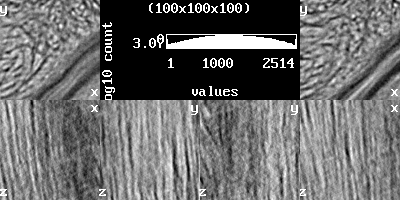scalar.gradient

Second step
Calculate the vector field and reduce it.

→ ImageXd

``` scalar.load stack.nii.gz // thread.max := 2 // here you can reduce the number of threads // scalar.diffusion 16 scalar.diffusion.adaptive 16 // if structure simple, do one step per 8 voxels vector.save "stack_vector.nii.gz" vector.save "stack_vector.txt" // for cloud2 newvector := vector.copyscale 0.1 vector.use newvector vector.save "stack_reduce.nii.gz" vector.save "stack_reduce.txt" ```

``` // scalar.diffusion.map map.pgm 16 // calculate diffusion map from one voxel with scan length at center point // scalar.diffusion.map map.pgm 16 <0,0,0> // calculate diffusion map from one voxel with scan length at center point // scalar.diffusion.map map.pgm 16 <10,10,10> // calculate diffusion map from one voxel with scan length at point <10,10,10> // scalar.diffusion.map map.pgm 16 <10,10,10> < -3,-3,-3> // calculate diffusion map from one voxel with scan length at point <7,7,7> // scalar.diffusion.map map.pgm 16 <0,0,0> < -3,-3,-3> // calculate diffusion map from one voxel with scan length at center point plus < -3,-3,-3> // scalar.diffusion.colormap map.ppm 16 ... // calculate diffusion map from one voxel with scan length at center point / ... see above // scalar.diffusion.dump dump.txt 16 ... // dump diffusion from one voxel with scan length at center point / ... see above // scalar.diffusion.histogram ... // calculate length histogram from one voxel at center point / ... see above // scalar.diffusion.sd ... // calculate sd's from one voxel at center point / ... see above // scalar.fullscan true // true = scan only full spheres, false = scan incomplete spheres // scalar.diffusion.method 5 // 1=stark2013 2=stark2014a 3=stark2014b 4=stark2015a 5=stark2015b(default) // algorithm for scalar.diffusion // stark2013 = only the single best vector // stark2014a = mean the two best vectors, if distance < r/2 // stark2014b = mean (same SD) best vectors // stark2015a = mean (same SD) best vectors (around diffusion.angle (rad)), only for diffusion.max (%) // stark2015b = mean the best vectors (around diffusion.angle (rad)), only for diffusion.max (%) // scalar.diffusion.max 0.25 // (%) // scalar.diffusion.angle 0.08726 // (rad) - 5*(pi/180) = 5° // scalar.diffusion 16 // calculate a vector field from scalar with voxel scan length // scalar.diffusion.x2 8 // calculate a half sized vector field from scalar with voxel scan length // scalar.diffusion.adaptive 16 // calculate a vector field from scalar with voxel scan length - only for scalar div 2 and if fails for subpixels // scalar.diffusion.adaptive.x2 8 // calculate a half sized vector field from scalar with voxel scan length - only for scalar div 2 and if fails for subpixels ```

Third step
Evaluation (with cloud2 please use *.txt).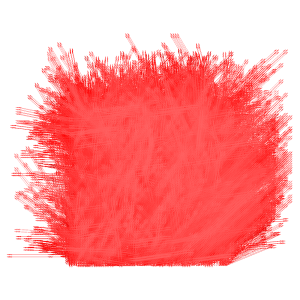stack_vector.txt (x,y view)stack_reduce.txt (x,y view)stack_reduce.txt (x,z view)

→ Cloud2

``` edit.global.mean.vectors ```stack_vector.txt (x,y view)

Third step / Advanced
Projection and Evaluation (with cloud2 please use stack_reduce.txt).

→ Cloud2

``` edit.vectors.norm transform.clear.z // transform.clear.x edit.local.mean.vectors ```stack.nii (x,y view)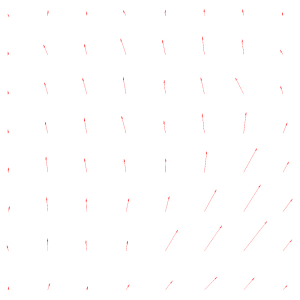stack_reduce.txt (x,y view)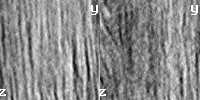stack.nii (y,z view)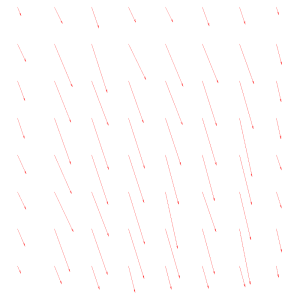stack_reduce.txt (y,z view)